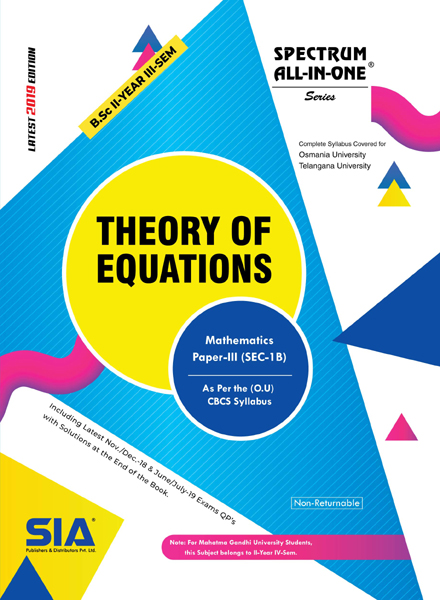•My WalletMy Order
•My Profile
•My Connections
•My Books
•My Videos
•My Tests
•My Calender
•My Messages
•My Shopping Cart
•My Orders
•Account Settings
•Help

# Book Details# Theory of Equations (O.U)

 Course Code : B.Sc II-III (O.U) Author : SIA PUBLISHERS University : Osmania University Regulation : 2019 Categories : Arts and Science Format :PDF (DRM Protected) Type : eBook

Rs.104 Rs.39 Rs.63% off

Description :

SYLLABUS

UNIT-1

Graphic representation of a polynomial – Maxima and minima values of polynomials – Theorems relating to the

real roots of equations – Existence of a root in the general equation – Imaginary roots – Theorem determining

the number of roots of an equation – Equal roots – Imaginary roots enter equations in pairs –Descartes rule

of signs for positive roots – Descartes rule of signs for negative roots.

UNIT-2

Relations between the roots and coefficients – Theorem – Applications of the theorem – Depression of an

equation when a relation exists between two of its roots – The cube roots of unity – Symmetric functions of

the roots–examples.

Note : Theory of Equations, B.Sc II-III (O.U)

##### Publisher Detail:

Publisher Name: SIA Publishers and Distributors (P) Ltd.

Contact Email Id: msultan@siaedugroup.com